Five mechanics

Five mechanics will make a car for some time. How many cars can 30 mechanics produce at the same time?

Result

n =  6

Solution:Leave us a comment of this math problem and its solution (i.e. if it is still somewhat unclear...):Be the first to comment!To solve this verbal math problem are needed these knowledge from mathematics:

Most natural application of trigonometry and trigonometric functions is a calculation of the triangles. Common and less common calculations of different types of triangles offers our triangle calculator. Word trigonometry comes from Greek and literally means triangle calculation.

Next similar examples:

1. Father and son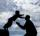Father is 44 years old, his son 16 years. Determine how many years ago was the father five times older than the son.
2. Hectoliters of waterThe pool has a total of 126 hectoliters of water. The first pump draws 2.1 liters of water per second. A second pump pumps 3.5 liters of water per second. How long will it take both pumps to drain four-fifths of the water at the same time?
3. Flood waterFlood waters in some US village meant that the homes had to evacuate 364 people. 50 of them stayed at elementary schools, 59 them slept with their friends and others went to relatives. How many people have gone to relatives?
4. Hr to minSue biked to school in 5/12 of an hour. How many minutes did it take her to ride to school?
5. HoursThe lesson lasts 45 minutes. For the week, students have 18 lesson hours. How many are the actual hours?
6. Clock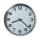How many hours are 15 days?
7. Train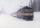The train passes part of the line for 95 minutes at speed 75 km/h. What speed would have to go in order to shorten the driving time of 20 minutes?
8. Monthly payments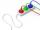Novákovi pay for electricity and monthly pays at the end of the year still bill 34 USD. Total per year pay 618 USD. What were their monthly payments?
9. Minutes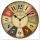Determine the difference in minutes: T1 = 2 3/20 h T2 = 2.3 h
10. Minute angle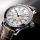Determine size of angle, which takes minute hand for 75 minutes.
11. Math classificationIn 3A class are 27 students. One-third got a B in math and the rest got A. How many students received a B in math?
12. Line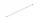How many parts of line divide 5 (different) points that lie on it?
13. CakesOn the bowl were a few cakes. Jane ate one-third of them, Dana ate a quarter of those cakes that remained. a) What part (of the original number of cakes) Dana ate? b) At least how many cakes could be (initially) on thebowl?
14. Addition of Roman numbersAdded together and write as decimal number: LXVII + MLXIV
15. Six te 2If 3t-7=5t, then 6t=
16. CagesHonza had three cages (black, silver, gold) and three animals (guinea pig, rat and puppy). There was one animal in each cage. The golden cage stood to the left of the black cage. The silver cage stood on the right of the guinea pig cage. The rat was in the
17. Circles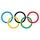For the circle c1(S1; r1=146 cm) and c2(S2; r2 = 144 cm) is distance of centers |S1S2| = 295 cm. Determine the distance between the circles.